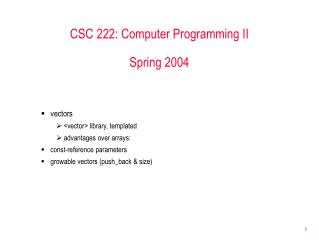DownloadDownload PresentationCSC 222: Computer Programming II Spring 2004

# CSC 222: Computer Programming II Spring 2004

Download Presentation## CSC 222: Computer Programming II Spring 2004

- - - - - - - - - - - - - - - - - - - - - - - - - - - E N D - - - - - - - - - - - - - - - - - - - - - - - - - - -
##### Presentation Transcript

1. CSC 222: Computer Programming IISpring 2004 • vectors • <vector> library, templated • advantages over arrays: • const-reference parameters • growable vectors (push_back & size)

2. Array disadvantages • size must be determined at compile-time • can't prompt the user for the size, then create it • means that you often waste lots of space • if run out of space, too bad! • no bounds checking on array accesses • can lead to unexpected results • parameter passing is very strange • when pass by value, function can still change the contents of the array • through a class definition, C++ provides for a "better array" • a vector is an array wrapped up in a class, with all the ugly details hidden • provides access to an indexable collection of data elements (all of same type) • in addition, provides other useful features not found in arrays • all of the above disadvantages of arrays are fixed using vectors!

3. Vectors • the vector class is defined in the <vector> library • when you declare a vector, you must specify the type and a size • the type is specified inside < > (vector is known as a templated class) • the size does not need to be known at compile-time #include <vector> // loads definition of the vector class using namespace std; vector<int> nums(100); // declares a vector of 100 ints // equiv. to int nums; for (int i = 0; i < 100; i++) { // vector elements are accessible via nums[i] = 0; // an index (same as with arrays) } vector<string> words(10); // declares a vector of 10 strings // equiv. to string words; int numGrades; cout << "How many grades are there? "; cin >> numGrades; vector<double> grades(numGrades); // declares a vector of numGrades // doubles (can't be done with arrays)

4. Size & resize • two useful member functions of the vector class are size & resize • size will return the amount of space allocated for the vector vector<int> nums(100); cout << nums.size() << endl; // displays 100 • resize expands (or shrinks) the vector, retaining the data nums.resize(200); // makes space for 100 more ints, // nums..nums is unchanged •  can read in and store an arbitrary number of values in pseudo-code: while (READ INPUT OK) { if (VECTOR IS FULL) { RESIZE THE VECTOR TO MAKE ROOM; } ADD INPUT TO VECTOR; INCREMENT ELEMENT COUNT; } • how big do you resize? • by 1? • by a constant amount? • double it?

5. Reverse array vs. Reverse vector #include <iostream> #include <string> using namespace std; const int MAX_SIZE = 100; int main() { string words[MAX_SIZE]; int numWords = 0; string input; while (numWords < MAX_SIZE && cin >> input) { words[numWords] = input; numWords++; } for (int i = numWords-1; i >= 0; i--) { cout << words[i] << endl; } return 0; } #include <iostream> #include <string> #include <vector> using namespace std; const int INITIAL_SIZE = 10; int main() { vector<string> words(INITIAL_SIZE); int numWords = 0; string input; while (cin >> input) { if (numWords == words.size()) { words.resize(2*words.size()); } words[numWords] = input; numWords++; } for (int i = numWords-1; i >= 0; i--) { cout << words[i] << endl; } return 0; } stops processing when array is full simply resizes vector when full, goes on

6. bounds checking & parameter passing • unlike arrays, vector access are checked for OOB int nums; vector<int> nums(100); for (int i=0; i<=100; i++) { for (int i=0; i<=100; i++) { nums[i] = 0; nums[i] = 0; } } RESULT: ??? RESULT: run-time error • unlike arrays, vector parameters behave as any other type void foo(vector<int> nums) void foo(vector<int> & nums) { { nums = 999; nums = 999; } } vector<int> numbers(10); vector<int> numbers(10); foo(numbers); foo(numbers); RESULT: nums is a copy of the vector, RESULT: nums is an alias for the numbers vector no change to numbers simultaneously changes numbers